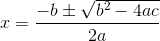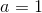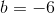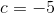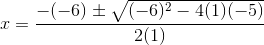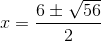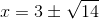# High School Math : Solving Quadratic Inequalities

## Example Questions

### Example Question #41 : Solving Quadratic Equations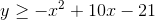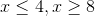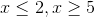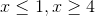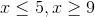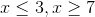Explanation:

Factor and solve.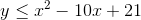Since the equation is less than or equal to, you know the inequality will be OR, not AND.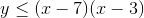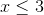or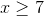### Example Question #42 : Solving Quadratic Equations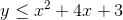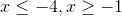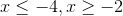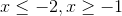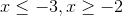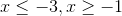Explanation:

Factor and solve. Since the sign is less than or equal to, we know the inequality will be OR, not AND.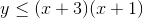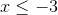or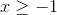### Example Question #43 : Solving Quadratic Equations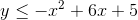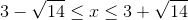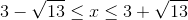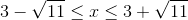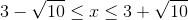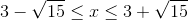Explanation:

Use the quadratic formula to solve.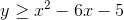Since the inequality is greater than or equal to, we know the inequality will be AND, not OR.